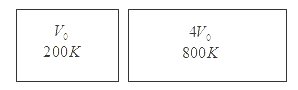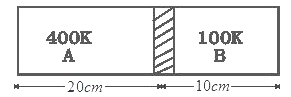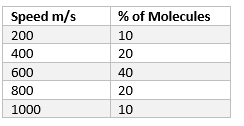# Important Problems on Kinetic theory of gases

Question 1.
Calculate the volume of 3 mole of an ideal gas at STP

At STP
Pressure P=105 Pascal
Temperature T=0° C=273 K
From ideal gas equation
PV=nRT (1)
where n is number of moles of gas. Thus from (1)
V=nRT/P
For 3 moles of an Ideal gas
V=3RT/P
R=8.3 J/mol-K
Now at STP
V=3 x 8.3 x 273/105
=6.79 x 10-2 m3

Question 2
Calculate the mass of 4 cm3 of nitrogen at STP

We know that
At STP
Pressure P=105 Pascal
Temperature T=0° C=273 K
From ideal gas equation
PV=nRT
where n is number of moles of gas
n=PV/RT
Substituting the values
n=1.76 x 10-4 Moles
We know that number of moles of the gas
N=Mass of gas in gm/Molecular mass in gm/moles
m=nM
=1.76 x 10-4 x 28
=4.94 mg

Question 3
Equal masses of air are filled and sealed in two container ,one of volume V0 and other of the volume 4V0.If the first vessel is maintained at 200K and other at 800K.Find the ratio of the pressure in the two containersGiven the mass of air filled in both the containers are equal
For gas in first container
T=200K,V=V0,N=n and P=P1
For gas in second container
T=800K,V=4V0 N=n since the mass are equal
Ideal gas equation for first gas
P1V0=nRx200---(1)
Ideal gas equation for Second gas
P2x4V0=nRx800---(2)
Dividing equation 1 and 2
P1:P2=1:1

Question 4
An glass bulb of volume 390 cc was sealed at a pressure of 2*10-3 mm of mercury at 25° C. Compute the number of the air molecules in the bulb .Avogadro number=6 x 1023 per mole, Density of mercury=13600Kg/m3
g=10 m/s2

We know that for any given height h ,Pressure P is
P=ρhg
For 2 x 10-3 mm of mercury
P=2 x 10-3 x 13600 x 10/1000
=.272Nm2
From Ideal gas equation
PV=nRT
or n=PV/RT
Substituting values
=4.2 x 10-8 moles
Number of air molecules=nNA
=2.57 x 1016

Question 5
A gas container has a walls that can bear maximum pressure of 2.2 x 106 pa.The container contains gas at 4 x 105 pa and 350K.If the container is steadily heated then neglecting change in volume, Calculate the temperature at which container will break?

Maximum pressure that the container can bear
Pm=2.2 x 106 pa---(1)
Ideal gas equation when the gas is in initial state
PV=nRT
4 x 105 xV=nRx350
Ideal gas equation when the gas reaches Pressure Pm
PmV=nRTm
2.2 x 106xV=nRxTm---(2)
Dividing equation 1 and 2
Tm=855.5K

Question 6
Density of an ideal gas at STP is 3 x 10-3 gm/cm3.Calculate the Molecular Weight of the gas

Given that
ρ=3 x 10-3 gm/cm3
Molecular weight of the gas is given as
M=nNa
and we know that ρ=m/V
Now PV=nRT
or PV=(m/M)RT
M=ρRT/P
At STP
Pressure P=105 Pascal
Temperature T=0° C=273 K
So substituting all the values
M=67.97gm/mole

Question 7
A vessel of volume V=30 L contains ideal gas at temperature 0° C. After the portion of gas has been let out, the pressure in the vessel decreased by ΔP=.78 atm(Temp remaining constant).Find the mas of the gas released. The gas density under normal condition is ρ=1.3 g/L

Let m1 mass of the gas before the gas is released
m2 mass of the gas after the gas is released
Thus the mass of gas released
Δm=m1-m2
From ideal gas equation
PV=nRT
Now before release
P1V=n1RT0
Now after release
P2V=n2RT0
where n1=m1/M and n2=m2/M
Number of moles of the gas before and after the release and V and T will remain same
(P1-P2)V=(n1-n2)RT0
=(m1-m2)RT0/M
or Δm=(P1-P2)VM/RT0---(1)
Also we know that
PV=(m/M)RT
M/RT0=ρ/P0---(2)
where P0=standard atmospheric pressure and T0=273K
From 1 and 2
Δm=ρVΔP/P0
Substituting all the values
Δm=30gm

Question 8
A vessel of volume V=20L contains a mixture of hydrogen and helium at T=20° C and Pressure P=2.0 atm.The mass of the mixture is equal to m=5g.Find the ratio of the mass of the hydrogen to that mass of the helium in the mixture. Given R=.082 L.atm.mol-1.K-1

Let the mixture contains:-
m1 mass of hydrogen
m2 mass of Helium
n1 moles of hydrogen
n2 moles of Helium
M1 Molecular mass of hydrogen
M2 Molecular mass of Helium

then m1=n1M1 and m2=n2M2
Also m=m1+m2
=n1M1 +n2M2 (1)
let n be the total no of moles in the mixture then
n=n1+n2
Now putting n1=n-n2 in 1 we get
n2=(m-nM1)/(M2-M1)
Similarly putting n2=n-n1 in 1 we get
n1=(nM2-m)/(M2-M1)
therefore
m1=M1[(nM2-m)/(M2-M1)]
Similarly
m2=M2[(m-nM1)/(M2-M1)]
so, ratio of m1 and m2 is
m1/m2=(M1/M2(nM2-m/m-nM1)
Now for hydrogen M1=2
for helium M2=4
Given m=5 gm
For mixture
PV=nRT
n=PV/RT
substituting the values
n=1.66
So m1/m2=.50

Question 9
Find the rms speed of the hydrogen molecules in a sample of hydrogen gas at 250K .Find the temperature at which rms speed is three times the speed at 250K

we know that
Vrms=√(3RT/M)---(1)
Now T=25oK
M=2 gm/Moles=.002Kg/mole
R=8.3J/mol-K
Vrms=1.76 x 103 m/s
Now we have to calculate the temperature at which rms becomes triple
Squaring equation 1
(Vrms)2=3RT/M
T=Vrms2M/3R
=(3 x 1.76 x 103) x.002/3 x 8.3
=2239K

Question 10
An ideal gas sample of .203 gm occupies 1000 cm3 at STP.Calculate the RMS speed of the molecules

Mass of sample=.203 gm
V=1000 cm3
At STP
Pressure P=105 Pascal
Temperature T=0°C=273K
Vrms=√(3P/ρ)
Now ρ=m/V
So
Vrms=√(3PV/m)
Substituting all the values
Vrms=1215.6 m/s

Question 11
The mean speed of the molecules of a hydrogen sample equals the mean speed of the molecules of helium sample. Calculate the ratio of temperature of hydrogen sample to the temperature of the helium sample.

(Vrms)H=(Vrms)He
if the TH and THe are respective temperature of the hydrogen and helium samples then
√(3RTH/2)=√(3RTHe/4)
or TH:THe=1:2

Question 12
Find the ratio of the mean speed of the hydrogen molecule to the mean speed of the oxygen molecules in a sample containing mixture of two gases

We know that
Vrms=√(3RT/M)
Where M is the molecular weight
(Vrms)H=√(3RTH/2)
(Vrms)O=√(3RTH/32)
so (Vrms)H:(Vrms)O=4:1

Question 13
A vessel is partitioned by a fixed diathermic separator. Different ideal gases are filled in the two parts. The rms speed of the molecules in the left part equals the mean speed in the right part. Calculate the ratio of the mass of the molecule in the left part to the mass of the molecule in the right part?

On left side rms speed of the molecule is given as
Vrms=√(3RT/m1)
On right side
Vmean=√(8RT/πm2)
Now
(Vrms)left=(Vmean)right
or m1:m2=1.18

Question 14
An air bubble of radius 3.00 mm is formed at the bottom of the 4 m deep river. Calculate the radius of the air bubble as it comes to the surface .Atmospheric pressure=105 Pa and density of the water=1000 kg/m3

Given that radius of bubble formed at bottom=3 mm=3 x 10-3 m
Depth of the river=4 m
Pressure on bubble at the bottom is
Pbottom=Pa + ρgH
Pa is atmospheric pressure
So, Pbottom=1.4 x 105 pa
Now from ideal gas equation
(PV)bottom=(PV)surface as temperature of water are same
at bottom and surface
1.4 x 105x(4/3)xπx(3 x 10-3)3=105x(4/3)xπxr3
r=3.35 mm

Question 15
Air is pumped into the tubes of a cycle at 1.5 atm pressure and volume of tube at this pressure is .002 m3.When one of the tubes get punctured volume of tube reduces to .0004 m3. Calculate the number of moles that leaked from the tube assuming that air is an ideal gas and temperature remains constant at 300K.

Air is filled in cycle tyres at pressure
P=1.5 atm=1.5 x 105 pa
and volume is V0=.002 m3
When tube get punctured, its volume becomes
V=.00004 m3
T=300K remains constant
Now from ideal gas equation
PV=nRT , where n is the no of moles
If n1 is no the moles before puncture
n1=PV0/RT
=.120 moles
After puncture, pressure of tube will become atmospheric pressure. If n1 is the no of moles after puncture
n2=PV/RT
=.0160 moles
Number of moles of gas leaked out
=n1-n2
=.120-.016
=.104 moles

Question 16
A tank attached to an air compressor contains 30L of air at temperature of 40° C and gauge pressure 5*105 pa. What is the mass of the air and what volume would it occupy at normal atmosphere pressure

Average molecular mas of air which is composed of many gases is M=28.8 gm/mol.
Gauge pressure is the amount of pressure in excess of atmospheric pressure. So total pressure is
P=5 x 105 pa +1 x 105 pa
=6 x 105 pa.
Expressing temperature in kelvin 40° C=313K.
Also express the volume in cubic meters 30L=30 x 10-3 m3
To find the number of moles
n=PV/RT
substituting all the values
n=6.91 mole
Mass of gas=nM=.199 kg
Volume at 1 atm and 0° C is
V=nRT/P=71.2 L

Question 17
Figure below shows two vessels A and B with rigid walls containing ideal gases. The pressure ,temperature and volume are PA,TA,V in vessel A and PB,TB,V in vessel B.Th. vessels are now connected through a small tube .Show that the pressure P and Temperature T satisfy
P/T=1/2[PA/TA + PB/TB]
when equilibrium is reachedIdeal gas equation for gases in vessel A and B are
PAV=nARTA
PBV=nBRTB
where nA and nB are no of molecules of gas in vessel A and B respectively
when equilibrium is reached, after connecting both the vessels gas equation becomes
P(2V)=(nA+nB)RT
or
P/T=(R/2V)(nA+nB)
P/T=(R/2V)[(PAV/RTA)+(PBV/RTB)] or
P/T=1/2[PA/TA + PB/TB]
which is the required relation

Question 18
Figure shows a cylindrical tube of length 30 cm partitioned by a tight fitting separator.The separator is weekly conducting and can freely slide along the tube. Ideal gases are filled in the two parts of the vessel. In the beginning, temp in two parts A and B are 400K and 100 K respectively. The separator slides to a momentary equilibrium position as shown in fig.Find the equilibrium position of the separator reached after the long timeLet, n1 and n2 are no of molecules of gas in left part and right part respectively
Ideal gas equation for gases in left and right part are
PV1=n1RT1
PV2=n2RT2
As cross-sectional area is same for both the portion
20AP=n1R x 400
10AP=n2R x 100
or n1:n2=1:2
If x is the distance of separator from left end and y from right end, then at common temperature
xAP=n1RT
yAP=n2RT
or x:y=n1:n2=1:2
so 2x=y ---(1)
now we know that
x+y=30 ----(2)
Solving 1 and 2
we get
x=10 and y=20

Assertion and Reason
a) Statement I is true ,statement II is true ,statement II is correct explanation for statement I
b) Statement I is true ,statement II is true ,statement II is not a correct explanation for statement I
c) Statement I is true,Statement II is false
d) Statement I is False,Statement II is True
Question 19
STATEMENT 1:The root mean square velocity of molecules of a gas having Maxwellian distribution of velocities is higher than then the most probable speed at any temperature
STATEMENT II:A very small number of molecules of as gas which possess very large velocities,increase the root mean square velocity without effecting the most probable speed.

(a)

Question 20
STATEMENT 1:Root mean square velocity of the gas does not change in a Isothermal process
STATEMENT 2:Isothermal process may be achieved by immersing the system in large reservoir and performing the process slowly

(b)

Question 21
STATEMENT 1:In an gas $v_{rms} > v_{mean}$
STATEMENT 2: In an gas $v_{rms} < v_{most probable}$

(c) Vrms=√(3RT/M)

Vm=√(8RT/πM)

V=√2RT/M

Vrms > Vm > V

Question 22
STATEMENT 1:Thermal conduction is the heat transfer that occurs in the direction of temperature decrease
STATEMENT 2:Thermal conduction is solids occurs due to vibrational energy of the molecules about the equilibrium position

(b) We know that Thermal conduction is the heat transfer that occurs in the direction of temperature decrease Also Thermal conduction is solids occurs due to vibrational energy of the molecules about the equilibrium position Both the statement are true ,but statement II is not a correct explanation for statement I

Question 23
Three identical cylinder $A_1$,$A_2$,$A_3$ contains equal moles of Helium,Nitrogen,Carbon dioxide at the same temperature
Match the column A to column B
Column A
A.Translational Energy is maximum in the cylinder
B.Total energy is minimum in the cylinder
C.Root mean square velocity is maximum in the cylinder
D.Mean speed will be minimum in the cylinder

Column B
P. $A_1$
Q. $A_2$
R. $A_3$
S. No appropriate match given

A->S
B->P
C->P
D->R
Molecular Mass
$M_{Helium} < M_{Oxygen} < M_{Carbon-dioxide}$

We know that Translational Energy is same for all molecules at the same temperature
Helium is monatomic gas while oxygen and carbon dioxide are diatomic and polyatomic gases so they posses rotation and vibrational energy also.So total energy is minimum for helium as root mean square velocity is given by $\sqrt {\frac {3RT}{M}}$ as M is least for helium so root mean square velocity will be maximum for Helium
Similarly Mean speed will be minimum for carbon dioxide

Question 24
Consider an ideal gas with following distribution of speeds(i) Calculate $V_{rms}$ and hence T. (m= 3.0 × 10-26 kg)
(ii) If all the molecules with speed 1000 m/s escape from the system, calculate new $V_{rms}$ and hence T.
$K_b= 1.38 \times 10^{-23}$

i. $V_{rms}^2= \frac {10 \times (200)^2 + 20 \times (400)^2 + 40 \times (600)^2 + 20 \times (800)^2 + 10 \times (1000)^2}{100}$
$V_{rms}=639 m/s$
Now
$\frac {1}{2} mv_{rms}^2 =\frac {3}{2}K_b T$
or
$T = \frac {1}{3K_b} mv_{rms}^2$
$T=296 K$
ii. $V_{rms}^2= \frac {10 \times (200)^2 + 20 \times (400)^2 + 40 \times (600)^2 + 20 \times (800)^2 }{90}$
$V_{rms}=584 m/s$
Now
$\frac {1}{2} mv_{rms}^2 =\frac {3}{2}K_b T$
or
$T = \frac {1}{3K_b} mv_{rms}^2$
$T=248 K$

Question 25
An ideal gas sample of .203 gm occupies 1000 cm3 at STP. Calculate the RMS speed of the molecules

Mass of sample=.203 gm
V=1000 cm3
At STP
Pressure P=105 pascal
Temperature T=0°C=273K
Vrms=√(3P/ρ)
Now ρ=m/V
So
Vrms=√(3PV/m)
Substituting all the values
Vrms=1215.6 m/s

Question 26
How will the rate of collision of a rigid diatomic molecules against the vessel will change ,if the gas is expanded adiabatically η times